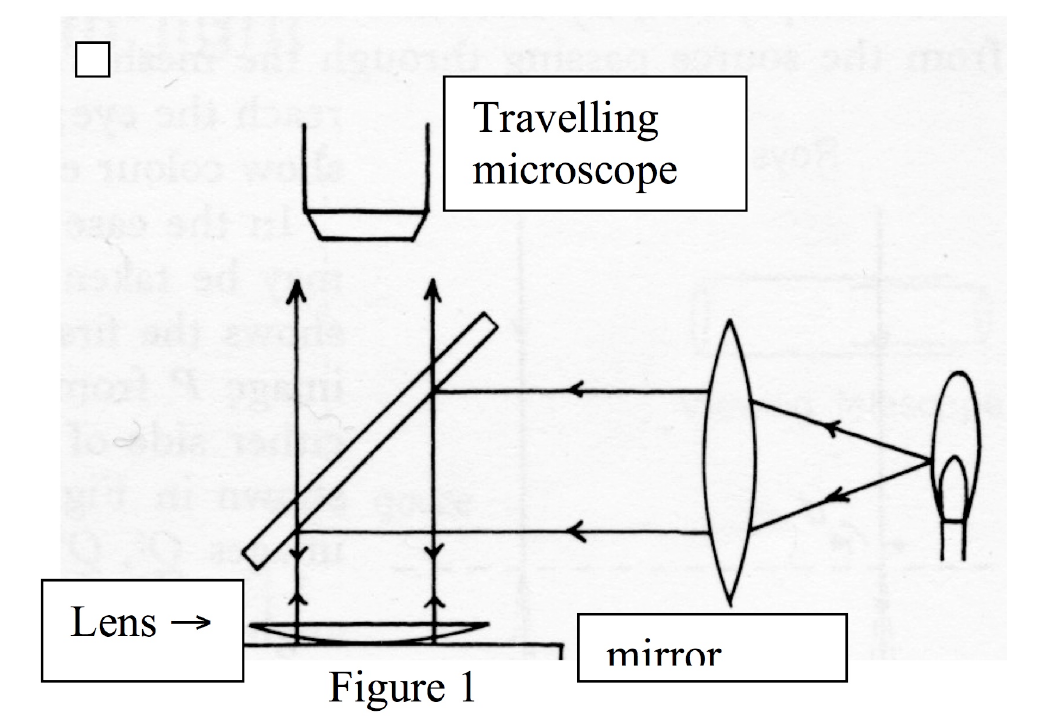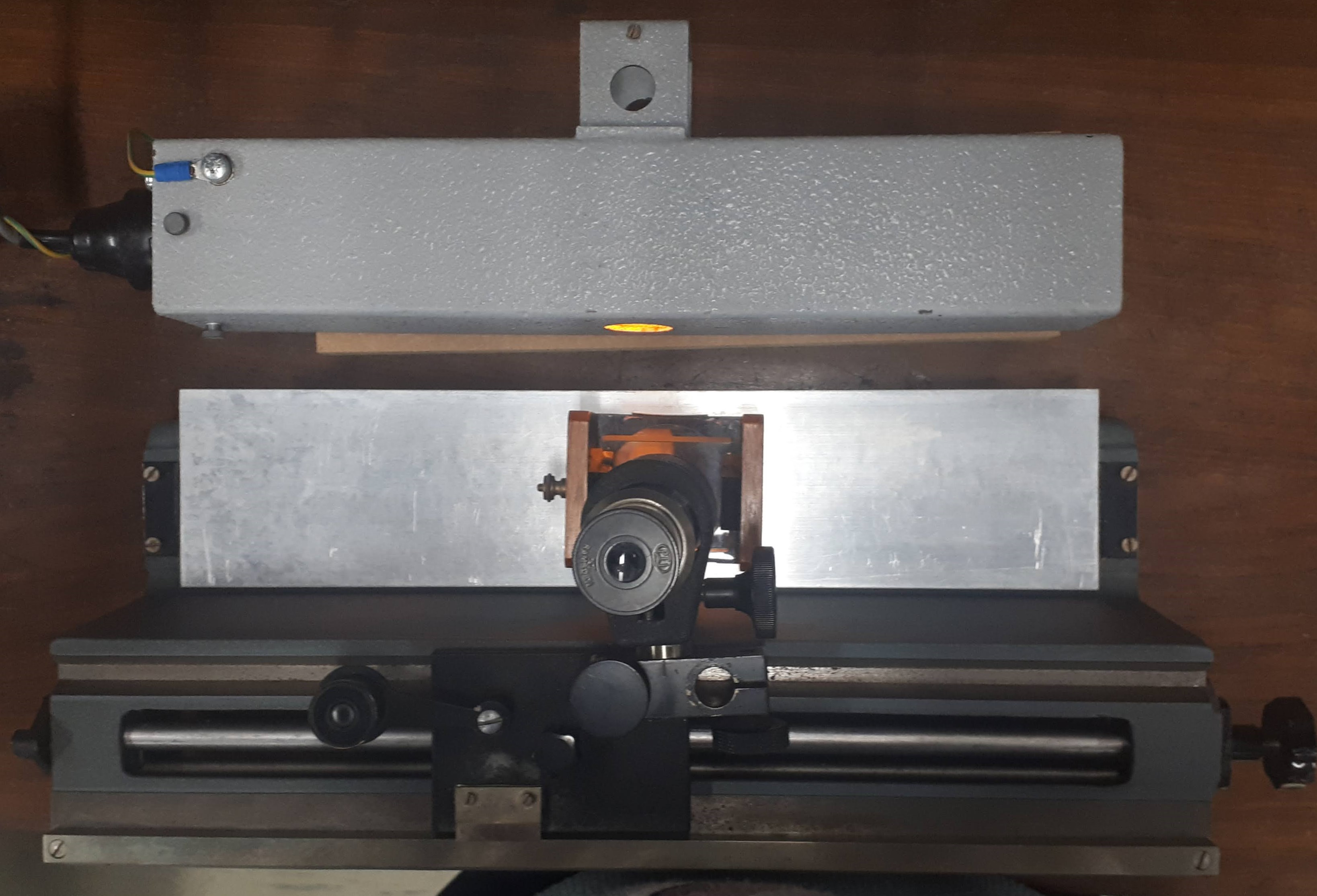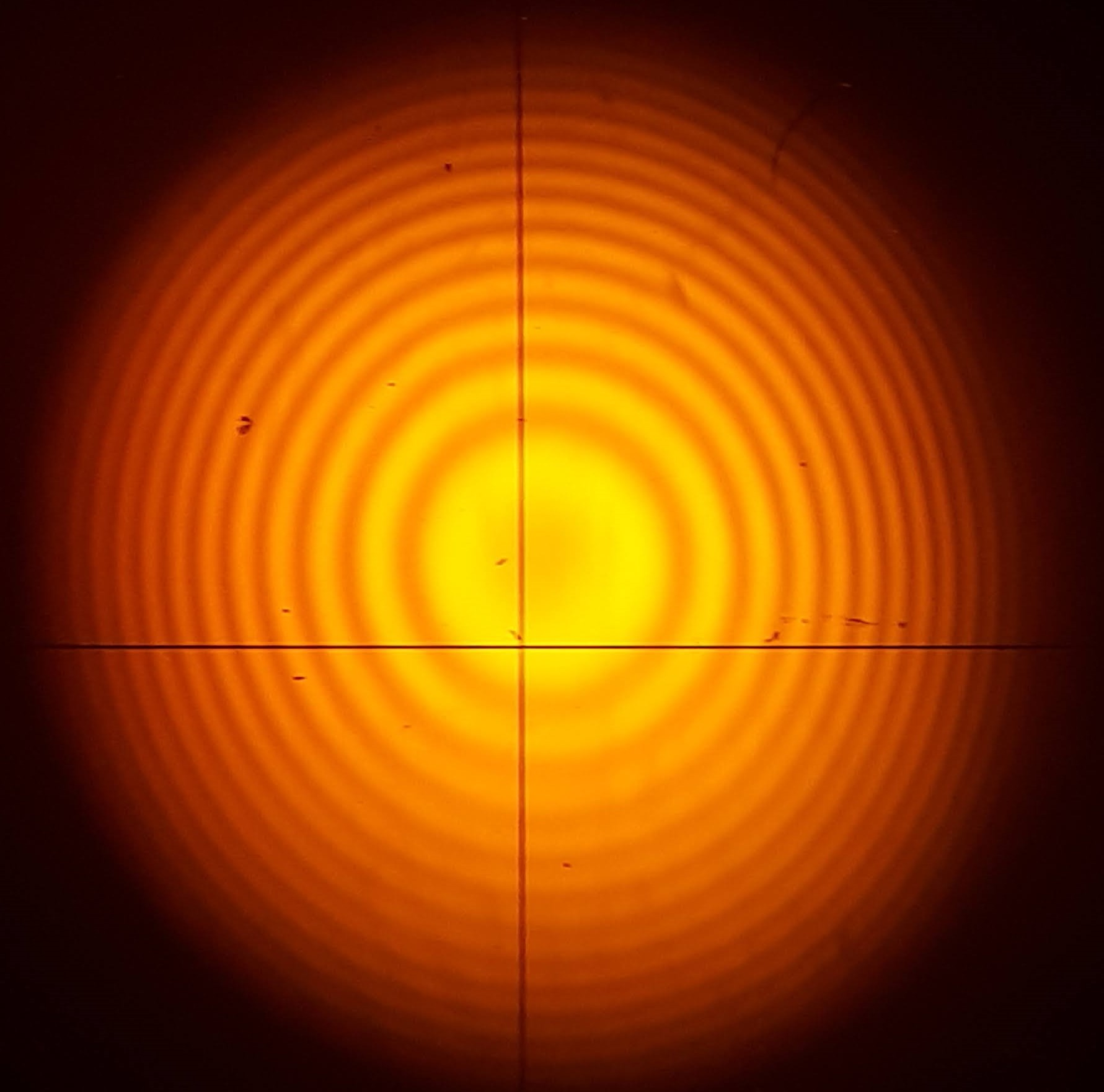# Newtons Rings

Newton's rings is a phenomenon in which an interference pattern is created by the reflection of light between two surfaces; a spherical surface and an adjacent touching flat surface. It is named after Isaac Newton, who investigated the effect. When viewed with monochromatic light (light consisting of one colour), Newton's rings appear as a series of concentric, alternating bright and dark rings centered at the point of contact between the two surfaces. When viewed with white light, it forms a concentric ring pattern of rainbow colors, because the different wavelengths of light interfere at different thicknesses of the air layer between the surfaces.

For this experiment a convex lens is placed on a reflecting surface. This is placed under a glass plate, angled at 45 degrees. The convex lens is the spherical surface and the reflecting surface is the flat surface. The glass plate is used to shine the light down on the lens and mirror. The light source, in this case a sodium lamp, is shone is horizaontally to the apparatus. It hits the back of the glass plate and partially reflects down to the lens and the mirror.The rings occur due to thin film interference which is where light waves which are reflected at the top and the bottom of a thin film interfere with one another. When light waves interefere they either add together, creating a brighter light, or subtract from one another, causing a dark fringe. To explore wave interference try this simulation: Wave Pulse Interference and Superposition. The thin film in this instance is the thin film of air between the reflecting surface and the lens. You can see this process very well in this simulation: Newtons Rings. You can also explore how colour changes the rings using this simulation: Newtons Rings

This is placed underneath a travelling microscope. A travelling microscope is a microscope lens assembly on a movable rail. The micriscope can be moved left and right along a scale so that the distance moved can be read on the scale. The students look through the microscope to see the Newtons rings. The microscope focus must be adjusted to see the rings correctly. The stidents can the move the microiscope left and right so they can count the rings. The ditance travelled is divided by the number of rings so that the average diameter of the rings can be calculated.The radius of curvature of the lens is then determined using a spherometer and that with the diameter of the rings can be used to get the wavelength of the light using:

D^2 = 4Rnλ

where D is the dimarer of the rings, R is the radiu s of curvatire of the lens, n is the refractive index of air and λ is the wavelength.

This experiment is then repeated after putting water inbetween the lens and the reflecting surface. The wavelngth of light is now known so the refractive index of water is determiend using the same equation.

You can try your hand at this experiment using this simulation/online lab:  Newton's Rings-Wavelength of light

## Department of Physics

Roinn na Fisice

Room 213 (Physics Office), 2nd floor, Kane Science Building, University College Cork, Ireland.,

Top